• //------------------------双边滤波----------------------- pcl::FastBilateralFilter<pcl::PointXYZRGB> fbf; fbf.setInputCloud(cloud); fbf.setSigmaS(5); // 设置双侧滤波器用于空间邻域/窗口的高斯...shell
• //------------------------双边滤波----------------------- pcl::BilateralFilter<pcl::PointXYZI> bf; bf.setInputCloud(cloud); bf.setSearchMethod(tree); bf.setHalfSize(0.1); // 设置高斯双边...shell
• //------------------------双边滤波----------------------- pcl::FastBilateralFilterOMP<pcl::PointXYZRGB> fbf; fbf.setInputCloud(cloud); fbf.setSigmaS(5); // 设置双侧滤波器用于空间邻域/窗口的...shell
• 双边滤波是一种非线性的滤波方法，能够保持边界清晰的情况下有效的去除噪声，它拥有类似相机里美颜的效果。 双边滤波之所以能够做到保边去噪的效果，是由于它的有两个核：空间域核和值域核，比高斯滤波只有一个值域...
双边滤波是一种非线性的滤波方法，能够保持边界清晰的情况下有效的去除噪声，它拥有类似相机里美颜的效果。
双边滤波之所以能够做到保边去噪的效果，是由于它的有两个核：空间域核和值域核，比高斯滤波只有一个值域核多了一个。
空间域核是由像素位置欧式距离决定的模板权值。
值域核是由像素值的差值决定的模板权值。
具体计算公式请直接百度，网络上有非常多的推导，理论。
可以用opencv自带的函数bilateralFilter()来实现双边滤波。
bilateraFilter(src, d, sigmaColor, sigmaSpace)
函数各参数的含义如下：
src：原图像；
d：像素的邻域直径；
sigmaColor：颜色空间的标准方差，一般尽可能大；
sigmaSpace：坐标空间的标准方差(像素单位)，一般尽可能小。
如上例，也可以写成：
cv2.bilateralFilter(src=img,d=13,sigmaColor=75,sigmaSpace=75) #参数名可省。
import cv2 as cv

t = cv.bilateralFilter(img, 13, 75, 75)
cv.imshow('org', img)
cv.imshow('res', t)
cv.waitKey(0)展开全文opencv
• 实现一个双边滤波（Bilateral Filter），并与高斯滤波比较保持图像边缘的效果，与cv::bilateralFilter比较效果和速度。 双边滤波：计算权重时同时考虑空间位置和像素颜色之差c++
• 双边滤波原理以及双边滤波算法的实现 双边滤波（Bilateral Filter）原理 双边滤波与高斯滤波器相比，对于图像的边缘信息能过更好的保存。其原理为一个与空间距离相关的高斯函数与一个灰度距离相关的高斯函数相乘。...
双边滤波原理以及双边滤波算法的实现

双边滤波（Bilateral Filter）原理

双边滤波与高斯滤波器相比，对于图像的边缘信息能过更好的保存。其原理为一个与空间距离相关的高斯函数与一个灰度距离相关的高斯函数相乘。

空间距离：指的是当前点与中心点的欧式距离。空间域高斯函数其数学形式为：其中（xi,yi）为当前点位置，（xc,yc）为中心点的位置，sigma为空间域标准差。

灰度距离：指的是当前点灰度与中心点灰度的差的绝对值。值域高斯函数其数学形式为：其中gray(xi,yi)为当前点灰度值，gray(xc,yc)为中心点灰度值，sigma为值域标准差。

对于高斯滤波，仅用空间距离的权值系数核与图像卷积后，确定中心点的灰度值。即认为离中心点越近的点，其权重系数越大。

双边滤波中加入了对灰度信息的权重，即在邻域内，灰度值越接近中心点灰度值的点的权重更大，灰度值相差大的点权重越小。此权重大小，则由值域高斯函数确定。

两者权重系数相乘，得到最终的卷积模板。由于双边滤波需要每个中心点邻域的灰度信息来确定其系数，所以其速度与比一般的滤波慢很多，而且计算量增长速度为核大小的平方。参数选择：

空间域sigma选取：其中核大小通常为sigma的6*sigma + 1。因为离中心点3*sigma大小之外的系数与中点的系数只比非常小，可以认为此之外的点与中心点没有任何联系，及权重系数为0.OpenCV中默认的计算公式也是如此，OpenCV参考文档内容如下：“对应高斯参数的 Gaussian sigma (标准差). 如果为零，则标准差由下面的核尺寸计算： sigma = (n/2 - 1)*0.3 + 0.8, 其中 n=param1 对应水平核,n=param2对应垂直核.”

值域sigma选取：另灰度差△g  =  abs（gray(xi,yi)- gray(xc,yc)），忽略常数的影响，因此其函数可以简化为：由的图像可知：已知 0≤△g≤255；

1）假设sigma = 255，当△g = 255时，系数为exp（-1） = 0.3679，当△g = 0时，系数为exp（-0）= 1.灰度最大点的系数与相差最小的灰度值系数之比为 0.3679.

2）假设sigma = 122.5，当△g = 255时，系数为exp（-4） = 0.0183，当△g = 0时，系数为exp（-0）= 1.灰度差最大点的系数与相差最小的灰度值系数之比为 0.0183.

结论：因为导数为，其增长速度为指数增长。

当simga较大时，灰度差最大值与最小值的系数在很小的一个范围之内，其比值较大。及灰度差较大的点，对于中心点也会有相应的较大的权值，此与双边滤波的保留边缘的初衷相违背。

当sigma较小时，灰度差最大值与最小值的系数在较大的一个范围之内，其比值很小，及灰度差较大的点，对应中心点仅有很小的权重。

综上分析可知：

Sigma越大，边缘越模糊，极限情况为simga无穷大，值域系数近似相等（忽略常数时，将近为exp（0）= 1），与高斯模板（空间域模板）相乘后可认为等效于高斯滤波。

Sigma越小，边缘越清晰，极限情况为simga无限接近0，值域系数近似相等（接近exp（-∞） =  0），与高斯模板（空间域模板）相乘后，可近似为系数皆相等，等效于源图像。

双边滤波算法实现：

在原理部分，从双边滤波的公式就可以得到该算法的实现途径。由于直接的编码实现上述过程，其时间复杂度为O(σs2) ，比较耗时，所以后来出现了一些改进算法，比较经典的有：论文《Fast O(1) bilateral ﬁltering using trigonometric range kernels》，提出了用Raised cosines函数来逼近高斯值域函数，并利用一些特性把值域函数分解为一些列函数的叠加，从而实现函数的加速[5，8]。
这里只对原始方法进行实现，从而有助于更加清楚的了解算法的原理。

matlab实现方法，这里也附一下核心代码：

第一种方法：

clc,clear all,close all;
ori=double(rgb2gray(ori))/255.0;
[width, height]=size(ori);
sigmaSpatial  = min( width, height ) / 30;
samplingSpatial=sigmaSpatial;
sigmaRange = ( max( ori( : ) ) - min( ori( : ) ) ) / 30;
samplingRange= sigmaRange;
output = bilateralFilter( ori, ori, sigmaSpatial, sigmaRange, ...
samplingSpatial, samplingRange );
figure,
subplot(1,2,1),imshow(ori,[]);title('input image');
subplot(1,2,2),imshow(output,[]);title('output image');

双边滤波函数：

function output = bilateralFilter( data, edge, sigmaSpatial, sigmaRange, ...
samplingSpatial, samplingRange )

if ~exist( 'edge', 'var' ),
edge = data;
end

inputHeight = size( data, 1 );
inputWidth = size( data, 2 );

if ~exist( 'sigmaSpatial', 'var' ),
sigmaSpatial = min( inputWidth, inputHeight ) / 16;
end

edgeMin = min( edge( : ) );
edgeMax = max( edge( : ) );
edgeDelta = edgeMax - edgeMin;

if ~exist( 'sigmaRange', 'var' ),
sigmaRange = 0.1 * edgeDelta;
end

if ~exist( 'samplingSpatial', 'var' ),
samplingSpatial = sigmaSpatial;
end

if ~exist( 'samplingRange', 'var' ),
samplingRange = sigmaRange;
end

if size( data ) ~= size( edge ),
error( 'data and edge must be of the same size' );
end

% parameters
derivedSigmaSpatial = sigmaSpatial / samplingSpatial;
derivedSigmaRange = sigmaRange / samplingRange;

paddingXY = floor( 2 * derivedSigmaSpatial ) + 1;
paddingZ = floor( 2 * derivedSigmaRange ) + 1;

% allocate 3D grid
downsampledWidth = floor( ( inputWidth - 1 ) / samplingSpatial ) + 1 + 2 * paddingXY;
downsampledHeight = floor( ( inputHeight - 1 ) / samplingSpatial ) + 1 + 2 * paddingXY;
downsampledDepth = floor( edgeDelta / samplingRange ) + 1 + 2 * paddingZ;

gridData = zeros( downsampledHeight, downsampledWidth, downsampledDepth );
gridWeights = zeros( downsampledHeight, downsampledWidth, downsampledDepth );

% compute downsampled indices
[ jj, ii ] = meshgrid( 0 : inputWidth - 1, 0 : inputHeight - 1 );

di = round( ii / samplingSpatial ) + paddingXY + 1;
dj = round( jj / samplingSpatial ) + paddingXY + 1;
dz = round( ( edge - edgeMin ) / samplingRange ) + paddingZ + 1;

% perform scatter (there's probably a faster way than this)
% normally would do downsampledWeights( di, dj, dk ) = 1, but we have to
% perform a summation to do box downsampling
for k = 1 : numel( dz ),

dataZ = data( k ); % traverses the image column wise, same as di( k )
if ~isnan( dataZ  ),

dik = di( k );
djk = dj( k );
dzk = dz( k );

gridData( dik, djk, dzk ) = gridData( dik, djk, dzk ) + dataZ;
gridWeights( dik, djk, dzk ) = gridWeights( dik, djk, dzk ) + 1;

end
end

% make gaussian kernel
kernelWidth = 2 * derivedSigmaSpatial + 1;
kernelHeight = kernelWidth;
kernelDepth = 2 * derivedSigmaRange + 1;

halfKernelWidth = floor( kernelWidth / 2 );
halfKernelHeight = floor( kernelHeight / 2 );
halfKernelDepth = floor( kernelDepth / 2 );

[gridX, gridY, gridZ] = meshgrid( 0 : kernelWidth - 1, 0 : kernelHeight - 1, 0 : kernelDepth - 1 );
gridX = gridX - halfKernelWidth;
gridY = gridY - halfKernelHeight;
gridZ = gridZ - halfKernelDepth;
gridRSquared = ( gridX .* gridX + gridY .* gridY ) / ( derivedSigmaSpatial * derivedSigmaSpatial ) + ( gridZ .* gridZ ) / ( derivedSigmaRange * derivedSigmaRange );
kernel = exp( -0.5 * gridRSquared );

% convolve
blurredGridData = convn( gridData, kernel, 'same' );
blurredGridWeights = convn( gridWeights, kernel, 'same' );

% divide
blurredGridWeights( blurredGridWeights == 0 ) = -2; % avoid divide by 0, won't read there anyway
normalizedBlurredGrid = blurredGridData ./ blurredGridWeights;
normalizedBlurredGrid( blurredGridWeights < -1 ) = 0; % put 0s where it's undefined
blurredGridWeights( blurredGridWeights < -1 ) = 0; % put zeros back

% upsample
[ jj, ii ] = meshgrid( 0 : inputWidth - 1, 0 : inputHeight - 1 ); % meshgrid does x, then y, so output arguments need to be reversed
% no rounding
di = ( ii / samplingSpatial ) + paddingXY + 1;
dj = ( jj / samplingSpatial ) + paddingXY + 1;
dz = ( edge - edgeMin ) / samplingRange + paddingZ + 1;

% interpn takes rows, then cols, etc
% i.e. size(v,1), then size(v,2), ...
output = interpn( normalizedBlurredGrid, di, dj, dz );


第二种方法：

%简单地说:
%A为给定图像，归一化到[0,1]的矩阵
%W为双边滤波器（核）的边长/2
%定义域方差σd记为SIGMA(1),值域方差σr记为SIGMA(2)

clc,clear all,close all;

I=double(I)/255;

w     = 5;       % bilateral filter half-width
sigma = [3 0.1]; % bilateral filter standard deviations

I1=bfilter2(I,w,sigma);

subplot(1,2,1);
imshow(I);
subplot(1,2,2);
imshow(I1)

滤波函数：

% Pre-process input and select appropriate filter.
function B = bfilter2(A,w,sigma)

% Verify that the input image exists and is valid.
if ~exist('A','var') || isempty(A)
error('Input image A is undefined or invalid.');
end
if ~isfloat(A) || ~sum([1,3] == size(A,3)) || ...
min(A(:)) < 0 || max(A(:)) > 1
error(['Input image A must be a double precision ',...
'matrix of size NxMx1 or NxMx3 on the closed ',...
'interval [0,1].']);
end

% Verify bilateral filter window size.
if ~exist('w','var') || isempty(w) || ...
numel(w) ~= 1 || w < 1
w = 5;
end
w = ceil(w);

% Verify bilateral filter standard deviations.
if ~exist('sigma','var') || isempty(sigma) || ...
numel(sigma) ~= 2 || sigma(1) <= 0 || sigma(2) <= 0
sigma = [3 0.1];
end

% Apply either grayscale or color bilateral filtering.
if size(A,3) == 1
B = bfltGray(A,w,sigma(1),sigma(2));
else
B = bfltColor(A,w,sigma(1),sigma(2));
end

灰色图像：

%%%%%%%%%%%%%%%%%%%%%%%%%%%%%%%%%%%%%%%%%%%%%%%%%%%%%%%%%%%%%
% Implements bilateral filtering for grayscale images.
function B = bfltGray(A,w,sigma_d,sigma_r)

% Pre-compute Gaussian distance weights.
[X,Y] = meshgrid(-w:w,-w:w);
%创建核距离矩阵，e.g.
%  [x,y]=meshgrid(-1:1,-1:1)
%
% x =
%
%     -1     0     1
%     -1     0     1
%     -1     0     1
%
%
% y =
%
%     -1    -1    -1
%      0     0     0
%      1     1     1
%计算定义域核
G = exp(-(X.^2+Y.^2)/(2*sigma_d^2));

% Create waitbar.
h = waitbar(0,'Applying bilateral filter...');
set(h,'Name','Bilateral Filter Progress');

% Apply bilateral filter.
%计算值域核H 并与定义域核G 乘积得到双边权重函数F
dim = size(A);
B = zeros(dim);
for i = 1:dim(1)
for j = 1:dim(2)

% Extract local region.
iMin = max(i-w,1);
iMax = min(i+w,dim(1));
jMin = max(j-w,1);
jMax = min(j+w,dim(2));
%定义当前核所作用的区域为(iMin:iMax,jMin:jMax)
I = A(iMin:iMax,jMin:jMax);%提取该区域的源图像值赋给I

% Compute Gaussian intensity weights.
H = exp(-(I-A(i,j)).^2/(2*sigma_r^2));

% Calculate bilateral filter response.
F = H.*G((iMin:iMax)-i+w+1,(jMin:jMax)-j+w+1);
B(i,j) = sum(F(:).*I(:))/sum(F(:));

end
waitbar(i/dim(1));
end

% Close waitbar.
close(h);


彩色图像：

%%%%%%%%%%%%%%%%%%%%%%%%%%%%%%%%%%%%%%%%%%%%%%%%%%%%%%%%%%%%%
% Implements bilateral filter for color images.
function B = bfltColor(A,w,sigma_d,sigma_r)

% Convert input sRGB image to CIELab color space.
if exist('applycform','file')
A = applycform(A,makecform('srgb2lab'));
else
A = colorspace('Lab<-RGB',A);
end

% Pre-compute Gaussian domain weights.
[X,Y] = meshgrid(-w:w,-w:w);
G = exp(-(X.^2+Y.^2)/(2*sigma_d^2));

% Rescale range variance (using maximum luminance).
sigma_r = 100*sigma_r;

% Create waitbar.
h = waitbar(0,'Applying bilateral filter...');
set(h,'Name','Bilateral Filter Progress');

% Apply bilateral filter.
dim = size(A);
B = zeros(dim);
for i = 1:dim(1)
for j = 1:dim(2)

% Extract local region.
iMin = max(i-w,1);
iMax = min(i+w,dim(1));
jMin = max(j-w,1);
jMax = min(j+w,dim(2));
I = A(iMin:iMax,jMin:jMax,:);

% Compute Gaussian range weights.
dL = I(:,:,1)-A(i,j,1);
da = I(:,:,2)-A(i,j,2);
db = I(:,:,3)-A(i,j,3);
H = exp(-(dL.^2+da.^2+db.^2)/(2*sigma_r^2));

% Calculate bilateral filter response.
F = H.*G((iMin:iMax)-i+w+1,(jMin:jMax)-j+w+1);
norm_F = sum(F(:));
B(i,j,1) = sum(sum(F.*I(:,:,1)))/norm_F;
B(i,j,2) = sum(sum(F.*I(:,:,2)))/norm_F;
B(i,j,3) = sum(sum(F.*I(:,:,3)))/norm_F;

end
waitbar(i/dim(1));
end

% Convert filtered image back to sRGB color space.
if exist('applycform','file')
B = applycform(B,makecform('lab2srgb'));
else
B = colorspace('RGB<-Lab',B);
end

% Close waitbar.
close(h);

转载地址：http://www.360doc.com/content/18/0807/12/58467320_776327983.shtml

转载地址：https://blog.csdn.net/piaoxuezhong/article/details/78302920

转载地址：https://blog.csdn.net/abcjennifer/article/details/7616663
展开全文• 双边滤波demo结构体参考 一. 线性滤波 1.1. 方框滤波 方框滤波是所有滤波器中最简单的一种滤波方式。每一个输出像素的是内核邻域像素值的平均值得到。 通用的滤波kernel如下： 这里是一个长宽分别为Kwidth和...
文章目录一. 线性滤波1.1. 方框滤波demo1.2. 均值滤波demo1.3. 高斯滤波demo二. 非线性滤波2.1. 中值滤波demo2.2. 双边滤波demo结构体参考
一. 线性滤波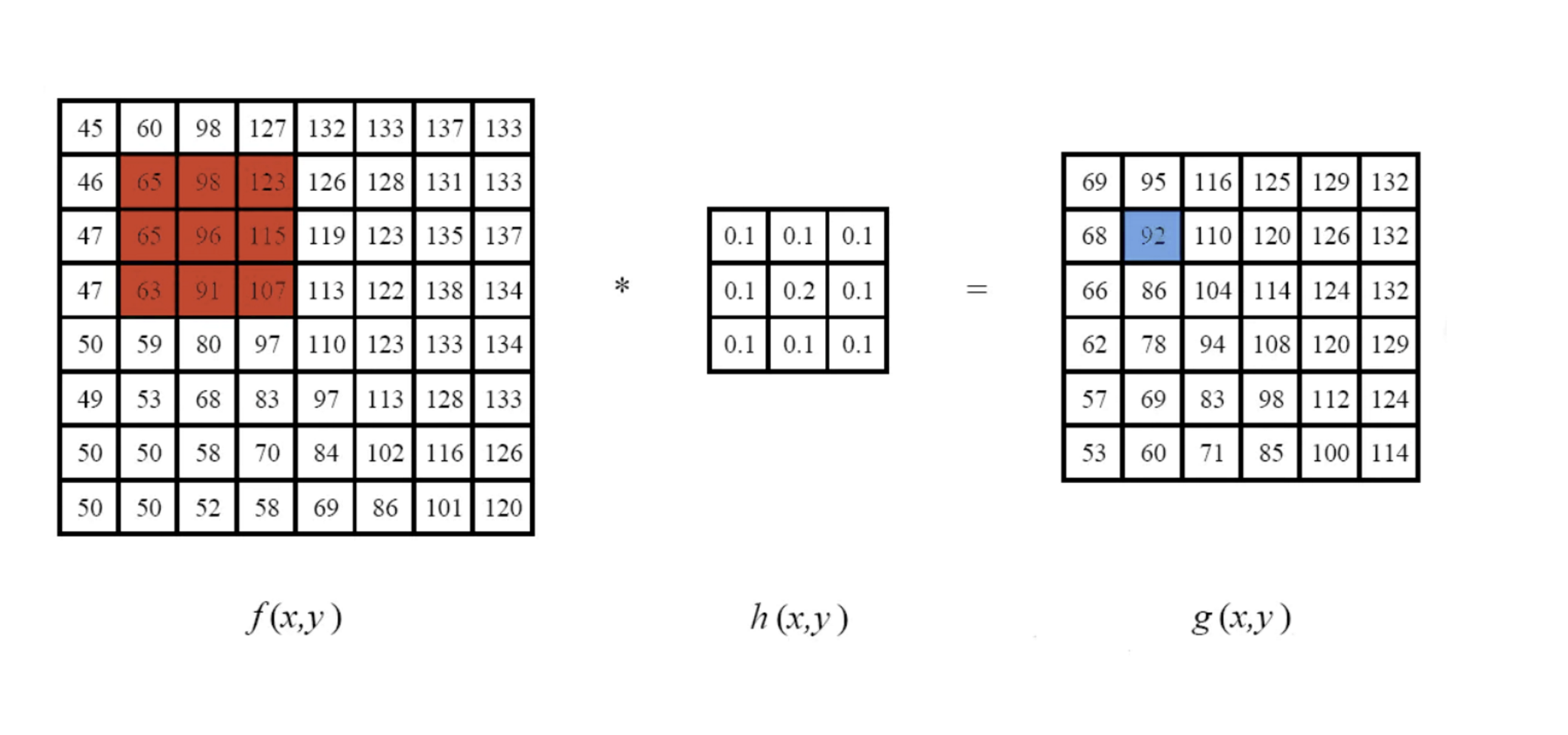1.1. 方框滤波
方框滤波是所有滤波器中最简单的一种滤波方式。每一个输出像素的是内核邻域像素值的平均值得到。
通用的滤波kernel如下：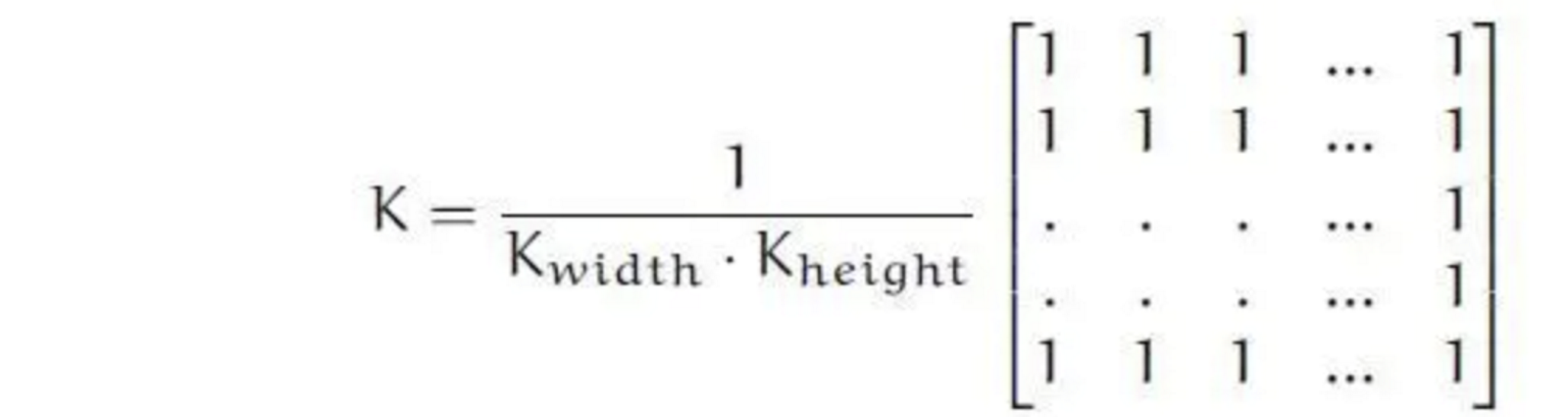这里是一个长宽分别为Kwidth和Kheight的窗口函数，在此区域内邻域中像素值叠加求平均即可求出位于kernel中心点像素的像素值。
/ ** @brief使用框过滤器模糊图像。
该函数使用内核对图像进行平滑处理：
未归一化的框式滤波器可用于计算每个像素邻域的各种积分特征，
例如图像导数的协方差矩阵（用于密集光流算法等）。
如果需要在可变大小的窗口上计算像素总和，请使用#integral。

@param src输入图像。
@param dst输出图像的大小和类型与src相同。
@param ddepth输出图像深度（使用src.depth（）时为-1）。
@param ksize模糊内核的大小。一般Size(w,h)来表示内核的大小。
@param 锚点（即被平滑的那个点）；默认值Point（-1，-1）表示锚点位于内核中心。
@param normalize标志，指定是否通过内核区域对其进行规范化。
@param borderType用于推断图像外部像素的边框模式，请参阅#BorderTypes
* /

CV_EXPORTS_W void boxFilter( InputArray src, OutputArray dst, int ddepth,
Size ksize, Point anchor = Point(-1,-1),
bool normalize = true,
int borderType = BORDER_DEFAULT );

boxFilter（）函数方框滤波所用的核为：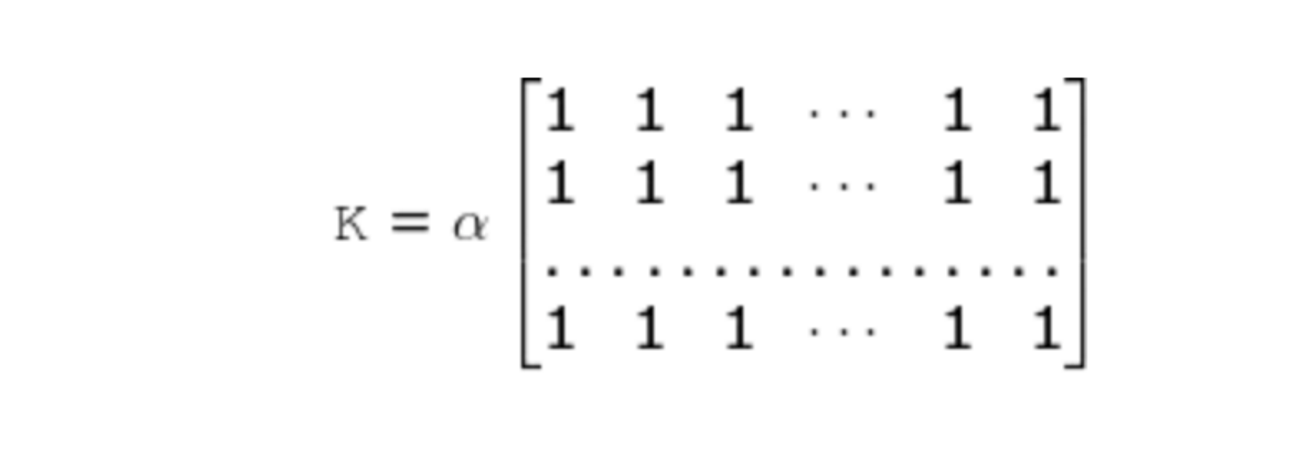其中：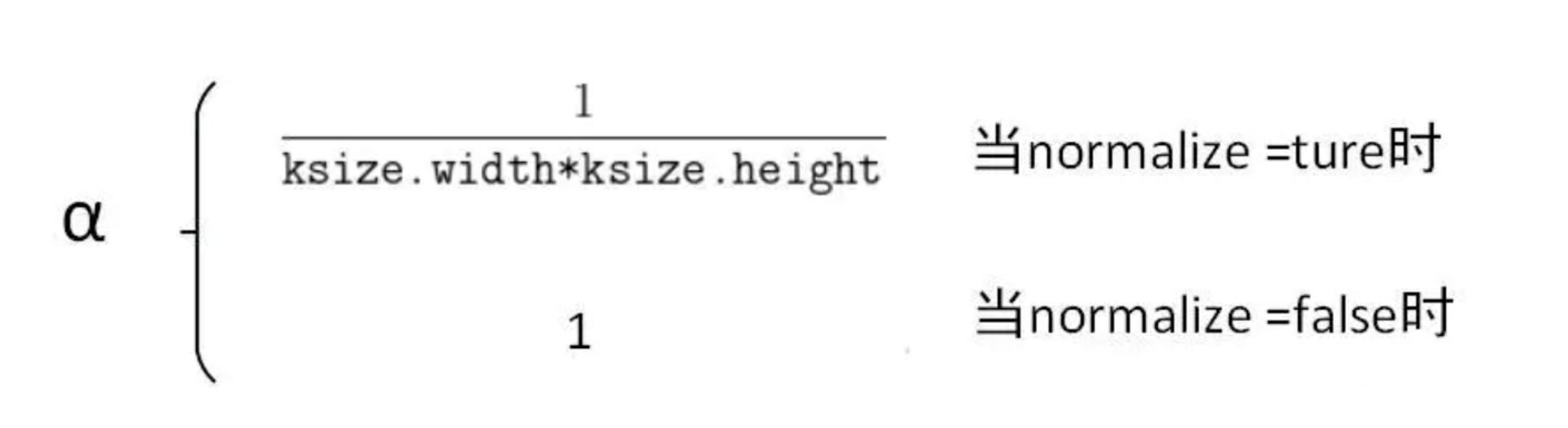当normalize=true的时候，方框滤波就变成了下面要说的的均值滤波。
demo
int MPT_test_boxFilter()
{
cv::Mat dst1, dst2, dst3, dst4;
cv::boxFilter(src, dst1, -1, cv::Size(5, 5), cv::Point(-1, -1), true);
cv::namedWindow("src", 0);
cv::imshow("src", src);
cv::namedWindow("方框滤波", 0);
cv::imshow("方框滤波", dst1);
cv::waitKey(0);
return 0;
}1.2. 均值滤波
均值滤波的原理非常简单，就是输出图像的每一个像素是核窗口内输入图像对应像素的像素的平均值( 所有像素加权系数相等)，其实说白了它就是归一化后的方框滤波。
但是均值滤波本身存在着固有的缺陷，即它不能很好地保护图像细节，在图像去噪的同时也破坏了图像的细节部分，从而使图像变得模糊，不能很好地去除噪声点。
/ ** @brief使用标准化框过滤器模糊图像。

该函数使用内核对图像进行平滑处理：
@param src输入图像； 它可以具有任意数量的通道，这些通道是独立处理的，但是深度应为CV_8U，CV_16U，CV_16S，CV_32F或CV_64F。
@param dst输出图像的大小和类型与src相同。
@param ksize模糊内核大小。
@param锚点； 默认值Point（-1，-1）表示锚点位于内核中央。
@param borderType用于推断图像外部像素的边框模式，请参阅#BorderTypes
*/
CV_EXPORTS_W void blur( InputArray src, OutputArray dst,
Size ksize, Point anchor = Point(-1,-1),
int borderType = BORDER_DEFAULT );

demo
//均值滤波
int MPT_test_blur() {
cv::Mat dst;
cv::blur(src, dst, cv::Size(5, 5));
cv::namedWindow("src", 0);
cv::imshow("src", src);

cv::namedWindow("均值滤波", 0);
cv::imshow("均值滤波", dst);
cv::waitKey(0);
return 0;
}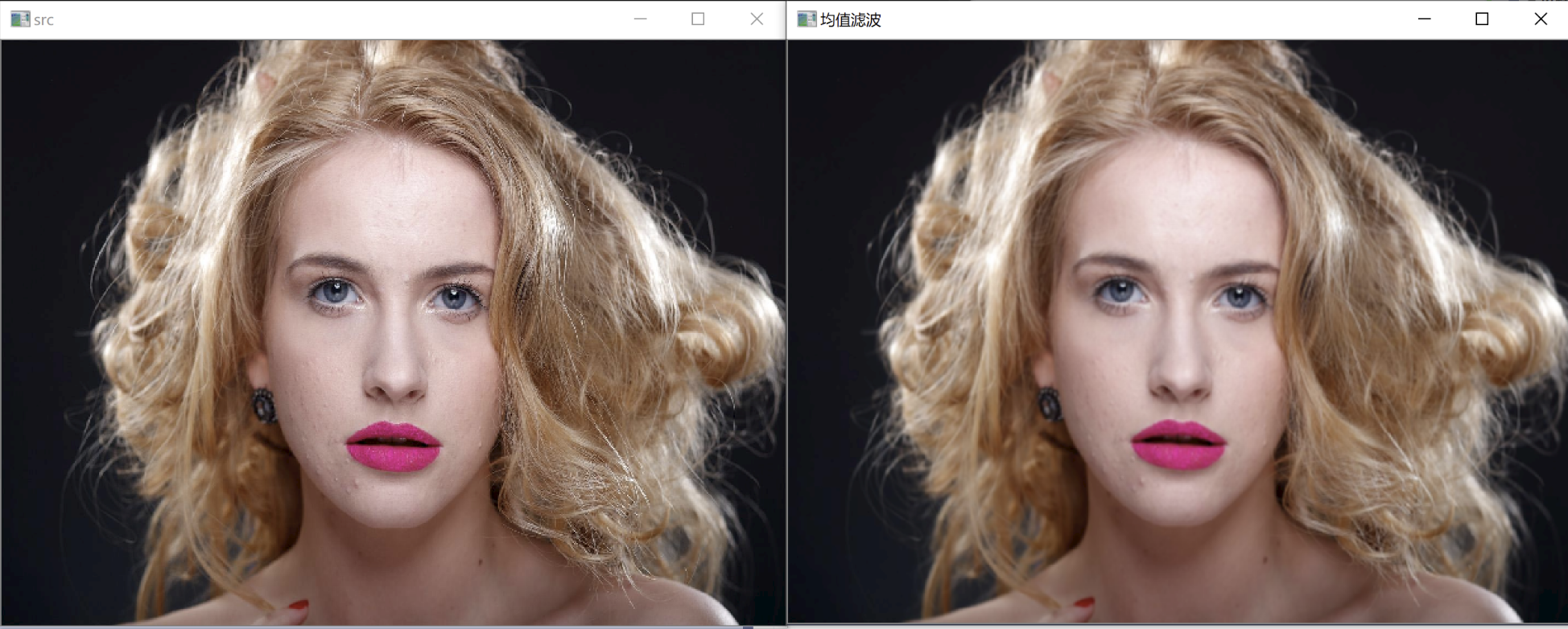1.3. 高斯滤波
图像的高斯模糊过程就是图像与服从二维正态分布的卷积核做卷积。由于正态分布又叫作高斯分布，所以这项技术就叫作高斯模糊。
图像与圆形卷积核做卷积将会生成更加精确的焦外成像效果。由于高斯函数的傅立叶变换是另外一个高斯函数，所以高斯模糊对于图像来说就是一个低通滤波操作。
高斯滤波器是一类根据高斯函数的形状来选择权值的线性平滑滤波器。高斯平滑滤波器对于抑制服从正态分布的噪声非常有效。
一维零均值高斯函数为：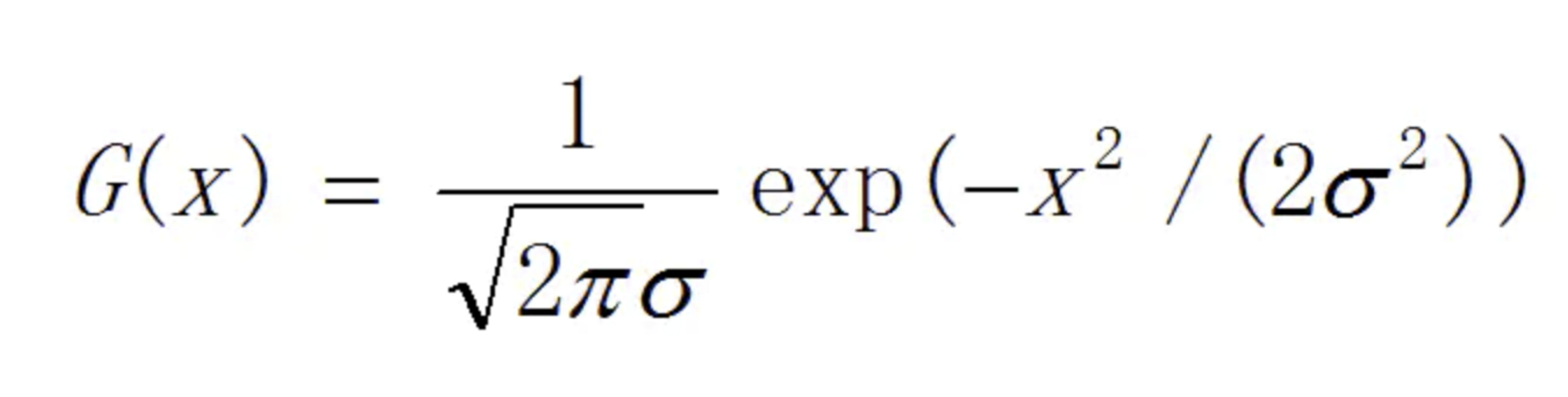其中，高斯分布参数σ决定了高斯函数的宽度。
对于二维图像来说，常用二维零均值离散高斯函数作平滑滤波器。
二维高斯函数为：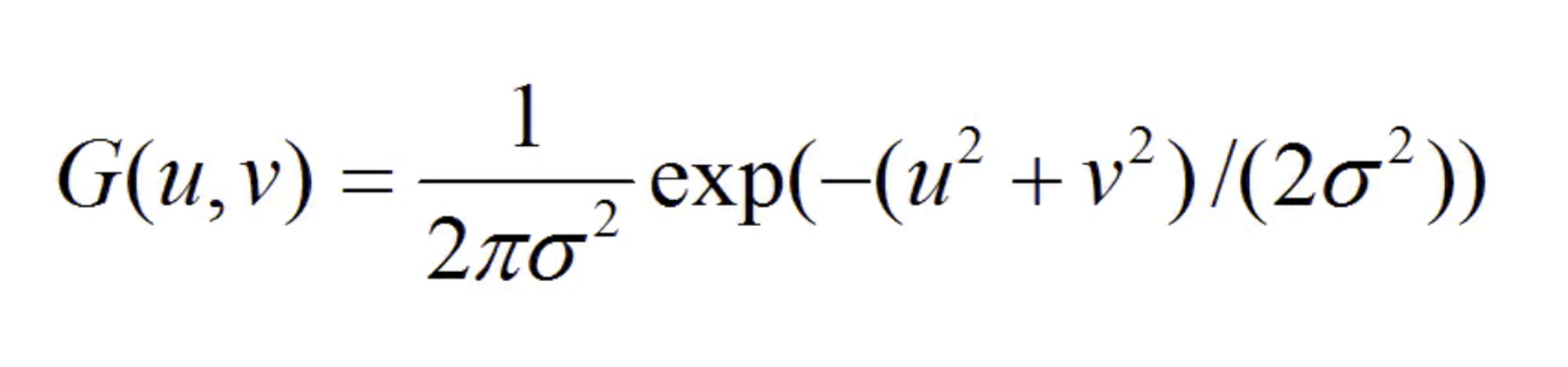/ ** @brief使用高斯滤镜模糊图像。

该函数将源图像与指定的高斯内核进行卷积。就地过滤是
支持的。

@param src输入图像；图像可以具有任意数量的经过处理的通道
但深度应为CV_8U，CV_16U，CV_16S，CV_32F或CV_64F。
@param dst输出图像的大小和类型与src相同。
@param ksize高斯内核大小。 ksize.width和ksize.height可以不同，但它们都必须为正数和奇数。或者，它们可以为零，然后根据sigma计算得出。
@param sigmaX X方向上的高斯核标准偏差。
@param sigmaY Y方向上的高斯核标准差；如果sigmaY为零，则将其设置为等于sigmaX；如果两个sigmas为零，则分别从ksize.width和ksize.height计算得出（有关详细信息，请参见#getGaussianKernel）；为了完全控制结果，而不考虑将来可能对所有这些语义的修改，建议指定所有ksize，sigmaX和sigmaY。
@param borderType像素外推方法，请参见#BorderTypes
*/

CV_EXPORTS_W void GaussianBlur( InputArray src, OutputArray dst, Size ksize,
double sigmaX, double sigmaY = 0,
int borderType = BORDER_DEFAULT );

demo
//高斯滤波
int MPT_test_GaussianBlur() {
cv::Mat dst;
cv::GaussianBlur(src, dst, cv::Size(5, 5), 0, 0);
cv::namedWindow("src", 0);
cv::imshow("src", src);

cv::namedWindow("高斯滤波", 0);
cv::imshow("高斯滤波", dst);
cv::waitKey(0);
return 0;
}二. 非线性滤波
非线性滤波器的原始数据与滤波结果是一种逻辑关系，即通过比较一定邻域内的灰度值大小来实现的。
2.1. 中值滤波
中值滤波原理：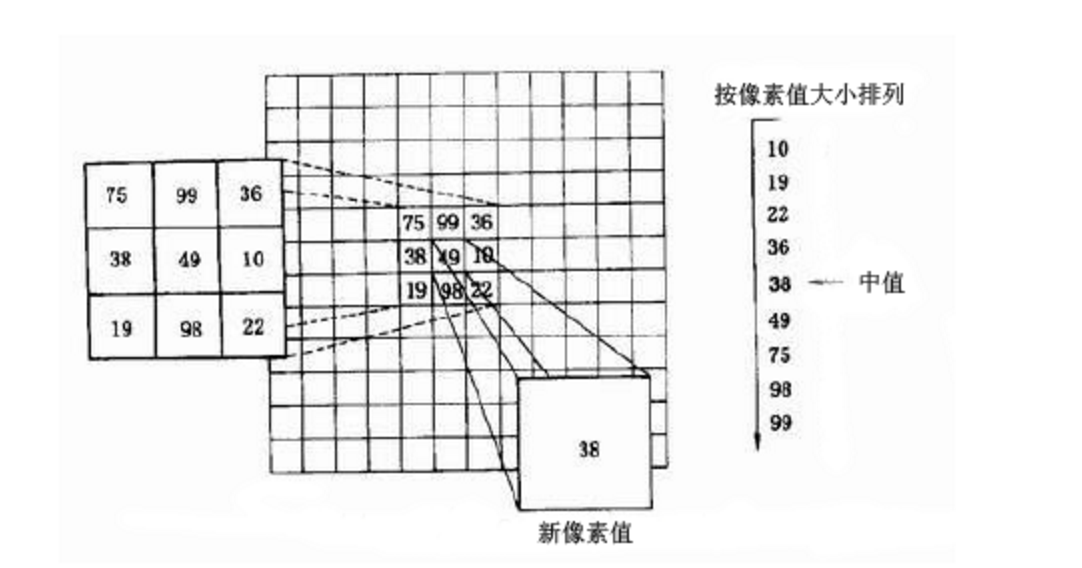简言之中值滤波就是把函数框（如图中的3 X 3）内的灰度值按顺序排列，然后中值取代函数框中心的灰度值。所以一般采用奇数点的邻域来计算中值，但如果像素点数为偶数，中值就取排序像素中间两点的平均值。
中值滤波在一定的条件下可以克服常见线性滤波器如方框滤波器、均值滤波等带来的图像细节模糊，而且对滤除脉冲干扰及图像扫描噪声非常有效，也常用于保护边缘信息, 保存边缘的特性使它在不希望出现边缘模糊的场合也很有用，是非常经典的平滑噪声处理方法。
但是中值滤波的缺点也很明显，因为要进行排序操作，所以处理的时间长，是均值滤波的5倍以上。
/ ** @brief使用中值滤镜模糊图像。
@note中值过滤器内部使用#BORDER_REPLICATE来处理边框像素，请参阅#BorderTypes

@param src输入1、3或4通道图像;当ksize为3或5时，
图像深度应为CV_8U，CV_16U或CV_32F，对于较大的光圈，只能为CV_8U。
@param dst目标数组，其大小和类型与src相同。
@param ksize孔径线性大小； 它必须是奇数且大于1，例如：3、5、7 ...
*/

CV_EXPORTS_W void medianBlur( InputArray src, OutputArray dst, int ksize );

demo
//中值滤波
int MPT_test_medianBlur() {
cv::Mat dst;
cv::medianBlur(src, dst, 9);
cv::namedWindow("src", 0);
cv::imshow("src", src);

cv::namedWindow("中值滤波", 0);
cv::imshow("中值滤波", dst);
cv::waitKey(0);
return 0;
}2.2. 双边滤波
双边滤波（Bilateral filter）是一种非线性的滤波方法，是结合图像的空间邻近度和像素值相似度的一种折衷处理，同时考虑空域信息和灰度相似性，达到保边去噪的目的。具有简单、非迭代、局部的特点。
双边滤波器的好处是可以做边缘保存（edge preserving），一般用高斯滤波去降噪，会较明显地模糊边缘，对于高频细节的保护效果并不明显。双边滤波器顾名思义比高斯滤波多了一个高斯方差sigma－d，它是基于空间分布的高斯滤波函数，所以在边缘附近，离的较远的像素不会太多影响到边缘上的像素值，这样就保证了边缘附近像素值的保存。但是由于保存了过多的高频信息，对于彩色图像里的高频噪声，双边滤波器不能够干净的滤掉，只能够对于低频信息进行较好的滤波。
下图是双边滤波的原理示意图：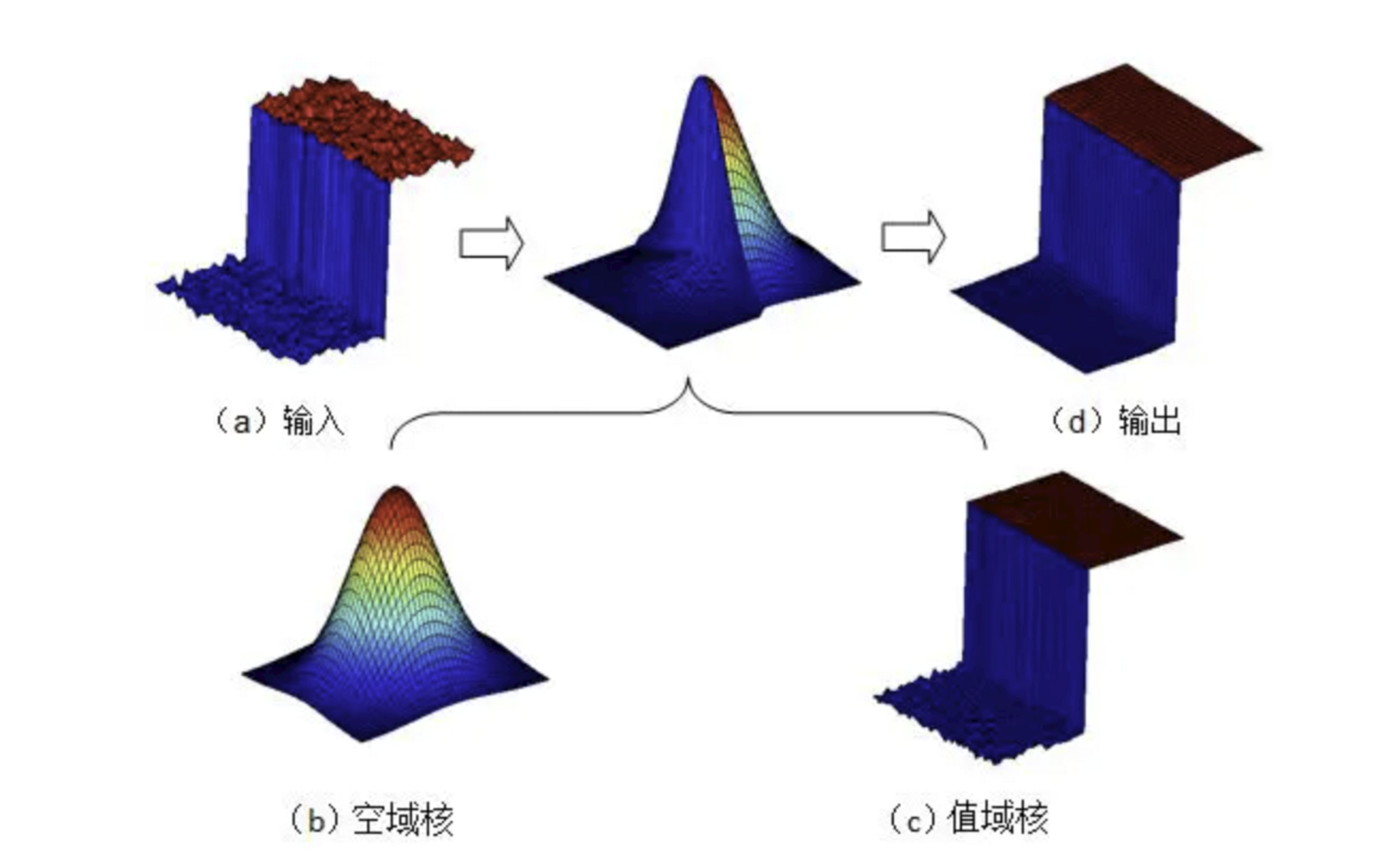在双边滤波器中，输出像素的值依赖于邻域像素值的加权值组合：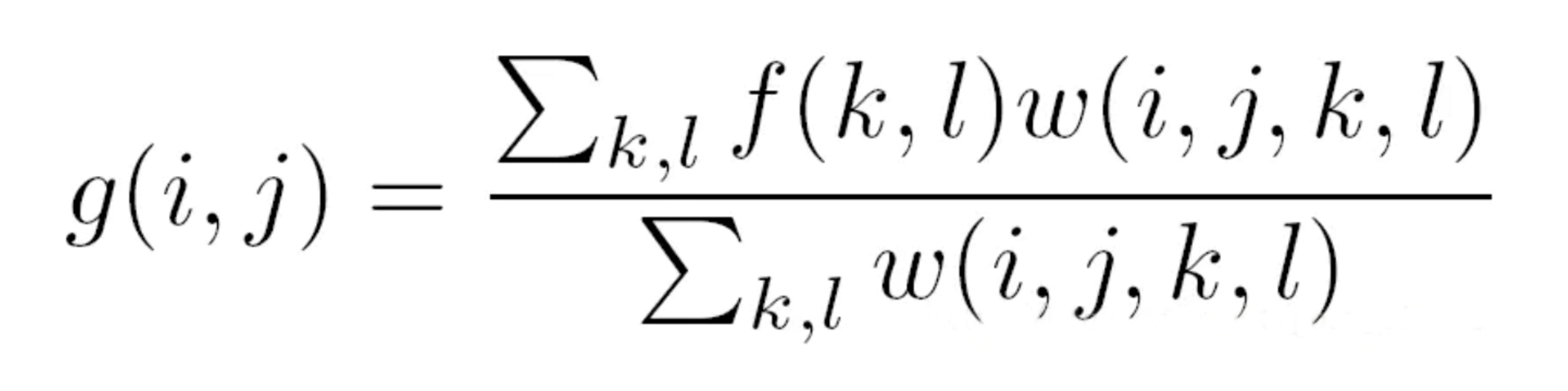而加权系数w(i,j,k,l)取决于空域核和值域核的乘积。
(i,j)，(k,l)分别指两个像素点的坐标。
其中空域核表示如下（如图）：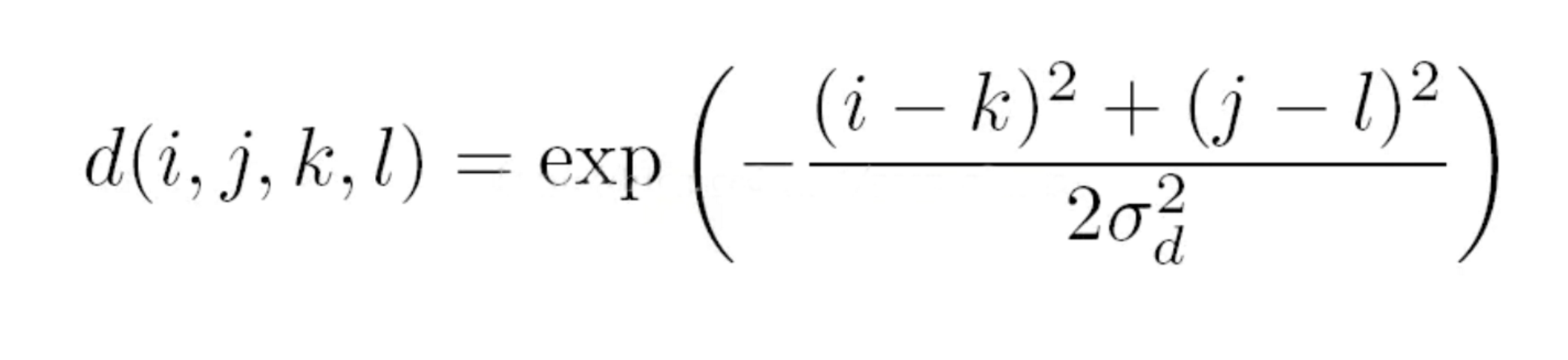值域核表示为：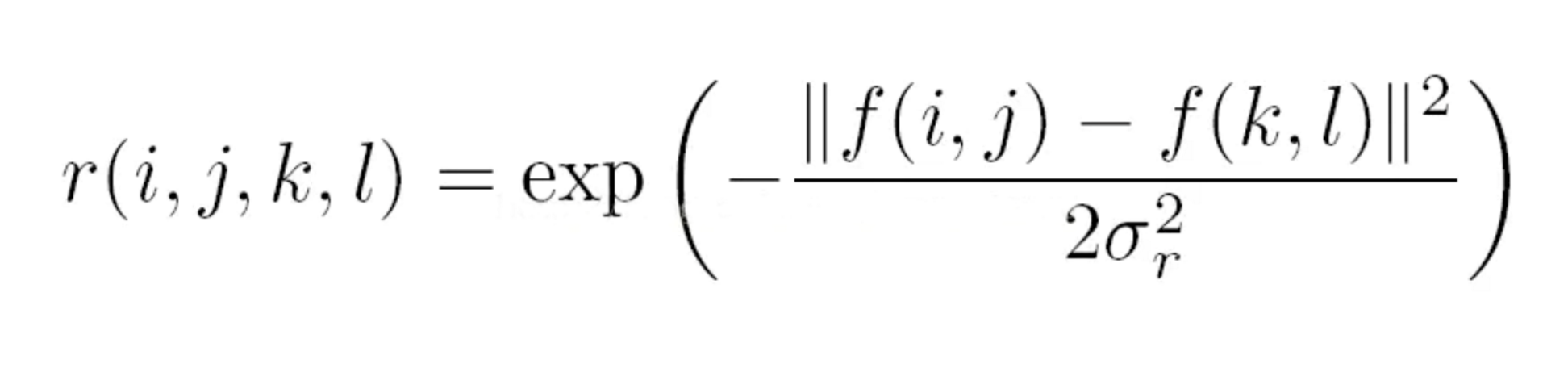两者相乘后，就会产生依赖于数据的双边滤波权重函数：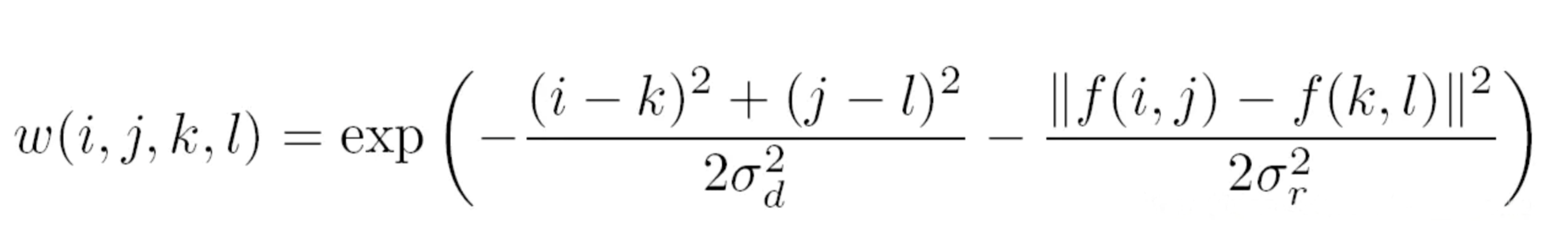d函数是根据像素距离选择权重，距离越近权重越大，这一点和方框滤波，高斯滤波方式相同。而r函数则是根据像素的差异来分配权值。如果两个像素值越接近，即使相距较远，也比差异大而距离近的像素点权重大。正是r函数的作用，使得边缘，即相距近但差异大的像素点的特性得以保留。
/ ** @brief将双边过滤器应用于图像。
可以在保持边缘相当清晰的同时很好地减少不必要的噪音。但是，与大多数过滤器相比，它非常慢。

_Sigma values_：为简单起见，您可以将2个sigma值设置为相同。如果它们很小（<10），则滤镜效果不大；
而如果它们很大（> 150），它们将具有非常大的效果。效果强，使图像看起来“卡通化”。

_Filter size_：大型滤镜（d > 5）非常慢，因此建议实时使用d = 5
应用程序，对于需要重噪声过滤的脱机应用程序，d = 9。

@param src  源8位或浮点，1通道或3通道图像。
@param dst  与src大小和类型相同的目标映像。
@param d    滤波期间使用的每个像素邻域的直径。如果它不是正值，
则从sigmaSpace计算得出。
@param sigmaColor 在色彩空间中过滤sigma。
这个参数的值越大，就表明该像素邻域内有更宽广的颜色会被混合到一起，产生较大的半相等颜色区域
@param sigmaSpace 在坐标空间中过滤sigma。
该参数的值越大，表示越远的像素就会相互影响，
只要它们的颜色足够接近即可（请参见sigmaColor）。
当d > 0时，它指定邻域大小，而不考虑sigmaSpace。
否则，d为与sigmaSpace成比例。
@param borderType 用于推断图像外部像素的边框模式，请参阅#BorderTypes

*/

CV_EXPORTS_W void bilateralFilter( InputArray src, OutputArray dst, int d,
double sigmaColor, double sigmaSpace,
int borderType = BORDER_DEFAULT );


demo
//双边滤波
int MPT_test_bilateralFilter() {
cv::Mat dst;
cv::bilateralFilter(src, dst, 25, 10.0, 25);
cv::namedWindow("src", 0);
cv::imshow("src", src);
cv::namedWindow("双边滤波", 0);
cv::imshow("双边滤波", dst);
cv::waitKey(0);
return 0;
}结构体参考
enum BorderTypes {
BORDER_CONSTANT    = 0, //!< iiiiii|abcdefgh|iiiiiii  with some specified i
BORDER_REPLICATE   = 1, //!< aaaaaa|abcdefgh|hhhhhhh
BORDER_REFLECT     = 2, //!< fedcba|abcdefgh|hgfedcb
BORDER_WRAP        = 3, //!< cdefgh|abcdefgh|abcdefg
BORDER_REFLECT_101 = 4, //!< gfedcb|abcdefgh|gfedcba
BORDER_TRANSPARENT = 5, //!< uvwxyz|abcdefgh|ijklmno

BORDER_REFLECT101  = BORDER_REFLECT_101, //!< same as BORDER_REFLECT_101
BORDER_DEFAULT     = BORDER_REFLECT_101, //!< same as BORDER_REFLECT_101
BORDER_ISOLATED    = 16 //!< do not look outside of ROI
};



展开全文opencv 图像处理 滤波 高斯滤波
• 双边滤波是基于高斯滤波的思想，在高斯滤波的基础上，加上图像像素值对中心像素的影响，两者共同影响中心像素的值。 高斯滤波： 权重大小取决于中心像素的灰度值和邻域像素的灰度值得差，当边缘有较大的灰度...
双边滤波是基于高斯滤波的思想，在高斯滤波的基础上，加上图像像素值对中心像素的影响，两者共同影响中心像素的值。

高斯滤波：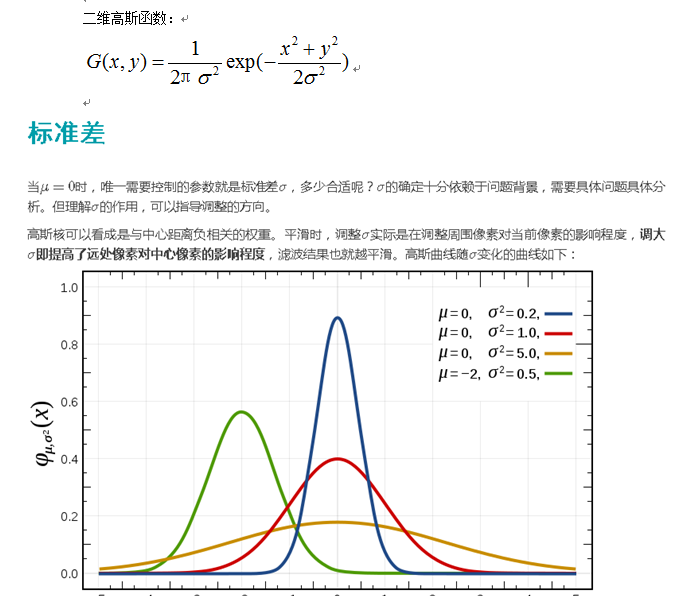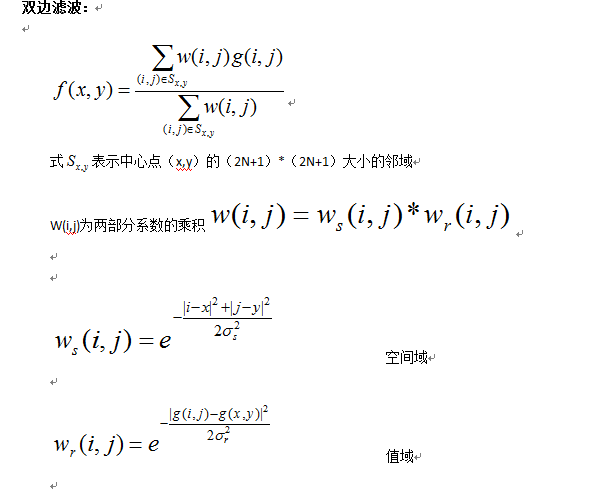权重大小取决于中心像素的灰度值和邻域像素的灰度值得差，当边缘有较大的灰度变化时，这个公式会使边缘和边缘另一边的区域生成较小的权值，与中心像素的灰度值类似的区域会生成较大的权值。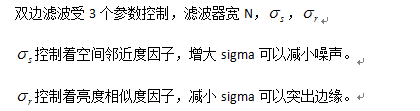下图能更好的理解双边滤波思想：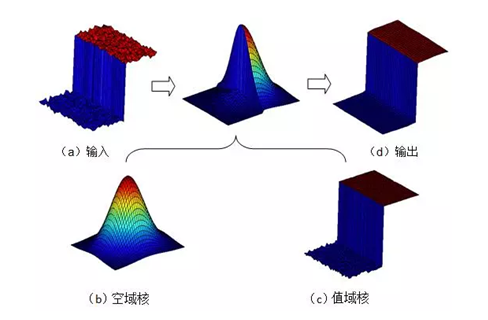基于双边滤波的锐化思想：

双边滤波在保存图像边缘的同时，滤除了图像噪声，具有保边去噪的效果。而同等sigma的高斯滤波只有去除噪声的效果。

用双边滤波后图像减去高斯滤波后图像，得到的图像即为图像的边缘。再与原图像叠加，有锐化效果。

展开全文图像锐化 图像处理...